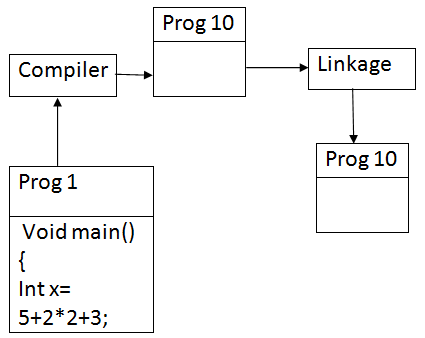Functions in C++ - Part II
Ratings:
(4)
Views:277## Functions in C++

What is a function in C++?

Function is a self-contained block which contain set of instructions.

It is a small program with in program

It performs operations.

Reusability: Write code once and use may time.

Modularity: Dividing programming instructions according their operations into small programs modules.

Simple: Easy to understand or road.

Efficiency :Functions increase efficiency of the program by avoiding redundancy code.

### Functions can be  divided into 2 parts in C++

Function Declaration

Function Definition.

### Function can be defined in 4 ways

Function with parameters with return type.

Function with parameters without return type.

Function without parameterswithout return type.

Function without parameter with return type.

### C++ Functions with out parameters  and without return type

This function return type is void and parameters are void.   void fun1(void) //return type as void { } void func1() { } func1() // default return type of any function is integer. { } #include <iostream.h> void draw-line() { cout<<end ; for (int i=1;i<=40;i++) cout<<”*”; } void Main() { draw-line(); cout<<”\n object oriented language”; draw-line(); }

When function is called execution control switched from calling function block to called function block.

After executing called function, execution control transfer to calling function block.

Memory for function is allocated on calling and deal located after executing.

### Function with parameters without return type

What is  a parameter in C++?

Parameters are local variables, which receive value from calling function.

The parameters which hold by function or called format parameters.

argumentsà sender, parameters àreceiver. printf(“%d”,a) => having 2 arguments printf()  à It is not valid because one argument must receive data à format argument, sender à actual argument.

If function required input from calling function to perform operation it require parameters.

C++ Supports 3 types of calling function

Pass by value.

pass by alias /reference.

Example #include<iostream.h> void draw-line(int length) { cout<<endl; for (int i=1;i<=length;i++) cout<<”*”; } void Main() { draw –line(20); cout<<”\n C++”; draw-line(40); cout<<”\n object oriented”; draw- line (20); } O/p: *********…..20times C++ *******----40 times Object oriented *******----20 times

### Pass by Value in C++

If function is called by sending value type variables or constant. In pass by value function parameter are declares of type value. Example of Pass by Value (pass by value) #include <iostream.h> void draw-line(int length,charch) { cout<<endl; for(int i=1;i<=length;i++) cout<<ch; } void main() { draw-line (20,’*’); cout<<“\n object oriented “; draw-line(20,’\$’); } O/p: ************* -- - - - 20 times ############# ------- 30 times object oriented \$\$\$\$\$\$\$\$-------- 20 times

### C++ Inline Functions

Every time function is called it takes a lot of extra time to executing a series of instructions like jump to the function ,pushing arguments to the stack and returning to the calling function.

When a function is small a substantial percentage of

To eliminate the cost of calls to small functions the solution is inline function

Inline Function

An inline function is declared with the key word inline

An inline function is a function that expanded inline when it is invoked

The compiler replaces the function call with corresponding function code

Syntax of Inline Functions inline function – header { function body }   Macros in ‘c’ language ( converts complex express it gives logical error) # define sum(a,b)a+b void main() { int r=sum(5,2)*sum*2,5); printf(“\n %d”,r); } o/p :Disadvantages of macros in C++

Macros are not functions

If macros are used in complex expression it leads to logic errors.

There is no type checking is done during complication

inline return type function-name (parameters) { statements;                                    * inline function is a function is }                                                        expand during compile time   Prog : # include<iostream.h> { inline void func1() { cout<<endl<<”This is inline funciton”; cout<<endl<<”This is expanded”; during compile time “; cout<<endl<<”This avoid control switching between fuctions”; } void main() { fun1(); func2(); }   Rules for inline expansion (situation where inline expansion doesn’t work)

If inline function contains loops (for loop, while loop, d-while) it does not expand as inline

If inline function having return type and return value.

inline void print() { for(int i=1;i<=10;i++) cout<<endl<<i; } void main() { Expl : In the above program point a function is not expand as inline becoz inline function contain loop(for) 3) if inline function are recursive, not expanded as online

• The above rules various from one compiler to another

eg : # include<iostream.h> inline int pow(int num, int p) { int res=1; for(int i=1; i<=1;i++) res= res * num; return res; } void main() { int r1=pow(5,2); cout<<endl<<r1; }

### Function with default arguments - - > (optional parameters)

C++ allows to write function with parameters by assigning values

Parameter having values doesn’t required to specify values in function call.

Default arguments are given from right to left

syntax : return type function – name (param, param,param=value,param=value,----) { statements; } eg : void funct1(int x, int y, intz=10) { cout<<endl<<x; cout<<endl<<y; cout<<endl<<Z; } void main() { fun1(30,40,50); func1(90,80) } void fun1(int x=10, int y) { int z=20 }

### prog :

void drawline1() { for(int i=1;i<=40;i++) cout<<”*”; } void draw line 2(char ch) { for(int i=1;i<=40;i++) cout<<ch; } void drawline (char ch=’x’) { void (int i=1;i<=y;i--) cout<<ch; } void main() { drawline(‘2’); drawline(); }

### Program

# include<iostream.h> void sample-inrest(float amt, int time, float rate=1.5) { float si=(amt * time *rate)/100; cout<<”\n simple interest is”<<si; } void main() { simple-intrest(5000,12); simple_intrest(7000,24,2.5); }